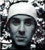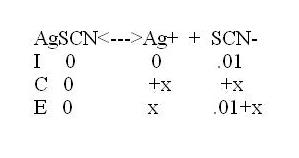# Trying to get my chem teacher fired

## Recommended Posts

This didn't make sense to me, and my chem teacher couldn't answer it either. She tried explaining to me for almost 15 minutes and still coulnd't come up with a solid and clear answer. The funny thing is that it was HER OWN problem:

Calculate the molar solubility product for AgSCN in pure water, and then in a

.01 molar solution of NaSCN.

Could someone please, for the love of Pete, help me solve this?

Yes, I am trying to get my chem teacher fired on account of her laziness, lack of chemistry knowledge, and her overall incompetence as an AP Chemistry teacher.

##### Share on other sites

AgSCN -----> Ag+ + SCN-

Ksp = [Ag+][sCN-]

but , i think theres not enough information to proceed !!!

is there any molar solubility given ???

##### Share on other sites

Is there any information that is missing? As chuinhen said, you can calculate the Ksp of AgSCN from its molar solubility. For the second question, you can do an ICE table (ICE stands for Initial Change Equilibrium), see example below.

If you know your Ksp, you can just substitute these into the concentrations,

Ksp=(x)(.01+x)=.01x+x^2

Then just solve for x. If I have made any mistakes, please correct me.##### Share on other sites

Wouldn't the presence of SCN- shift the equilibrium to the left and, thus, wouldn't the x's would be negative for Ag+ and SCN-?

btw, I'm trying to post as many of my chem teacher's stupid questions as possible as proof that she is incapable of teaching college chemistry.

##### Share on other sites
Wouldn't the presence of SCN- shift the equilibrium to the left and' date=' thus, wouldn't the x's would be negative for Ag+ and SCN-?

btw, I'm trying to post as many of my chem teacher's stupid questions as possible as proof that she is incapable of teaching college chemistry.[/quote']

Nope. The I.C.E. Table begins with NOTHING dissociated. So initially you have zero Ag+ in solution and zero -SCN in solution. (That's true when you have just AgSCN there). If you have the mixture of AgSCN and -SCN ions then your starting concentration of -SCN is whatever the molarity of your -SCN is. Initially, there is no -SCN from the silver thiocyanate and no silver ions because initially they are not dissociated. During the change portion of it, the ions dissociate and so you get +x moles of Ag+ and +x moles of -SCN thanks to the dissociation of AgSCN. At equillibrium, you get your starting concentrations of Ag+ and -SCN in addition to the change. Therefore, once you calculate the value of x you'll be able to caluclate the final concentrations.

##### Share on other sites

Another one of my teacher's questions that she herself couldn't answer:

Put the following in order from most ACIDIC to most BASIC. Assum aqueous solutions:

KCN, KOH, KBR, CH3COOH, NH4CN, NH4BR

I put them in the following order, but my answer was different from my teacher's:

NH4BR, CH3COOH, KBR, NH4CN, KCN, KOH

I argued my answer based on information she gave us in a lecture, so if anyone thinks my answer is wrong, my teacher is to blame.

This is the order my teacher wrote:

CH3COOH, NH4Br, NH4CN KBr, KCN, KOH

When I showed her her own power point presentation that she used for this chapter, she couldn't disprove my answer and so I don't know which answer is the correct one. Can someone clarify?

Here is the power point presentation my teacher used for this chapter if anyone cares to see it (I think there are numerous flaws in it):

http://www.mccsc.edu/~jpresley/apchp15.ppt

##### Share on other sites
Nope. The I.C.E. Table begins with NOTHING dissociated. So initially you have zero Ag+ in solution and zero -SCN in solution. (That's true when you have just AgSCN there). If you have the mixture of AgSCN and -SCN ions then your starting concentration of -SCN is whatever the molarity of your -SCN is. Initially, there is no -SCN from the silver thiocyanate and no silver ions because initially they are not dissociated. During the change portion of it, the ions dissociate and so you get +x moles of Ag+ and +x moles of -SCN thanks to the dissociation of AgSCN. At equillibrium, you get your starting concentrations of Ag+ and -SCN in addition to the change. Therefore, once you calculate the value of x you'll be able to caluclate the final concentrations.

Funny, because our teacher told us EXPLICITLY that because of the initial presence of SCN- would force us to do the following (and this is what our teacher wrote on the board):

AgSCN: initial = 0, change = +x, equilibrium = x

Ag+: i = 0, c = -x, e = -x

SCN-: i = .01, c = -x, e = .01 - x

##### Share on other sites

The Ka for the NH4+ ion is only 5.6E-10 so it is most definitely not a stronger acid than acetic acid is. If everything was in equal concentrations, this is how I would arrange it based on the Ka's and Kb's of the salts.

CH3COOH, NH4Br, KBr, NH4CN, KCN, KOH

The cyanide ion (CN-) is actually a pretty strong base due to it's very small Ka value. Therefore, the pH of a 1.0 molar solution would be around 11 or 12. This would be higher than the NH4CN solution because the combination of NH4+ and CN- ions kind of buffer each other out so you get a pH less than 10. KBr is perfectly neutral as it's the salt of a strong acid and strong base. NH4Br is slightly acidic due to the fact that the NH4+ ion is a very weak base, and CH3COOH is the most acidic due to it's relatively large Ka value.

##### Share on other sites
Funny' date=' because our teacher told us EXPLICITLY that because of the initial presence of SCN- would force us to do the following (and this is what our teacher wrote on the board):

AgSCN: initial = 0, change = +x, equilibrium = x

Ag+: i = 0, c = -x, e = -x

SCN-: i = .01, c = -x, e = .01 - x[/quote']

How can you have a negative concentration of the silver ion?If your initial concentration is zero, then the only way it can change is to move upward, or not change at all.

##### Share on other sites
How can you have a negative concentration of the silver ion?If your initial concentration is zero, then the only way it can change is to move upward, or not change at all.

my thoughts exactly

##### Share on other sites
Is there any information that is missing? As chuinhen said' date=' you can calculate the Ksp of AgSCN from its molar solubility. For the second question, you can do an ICE table (ICE stands for [b']I[/b]nitial Change Equilibrium), see example below.

If you know your Ksp, you can just substitute these into the concentrations,

Ksp=(x)(.01+x)=.01x+x^2

Then just solve for x. If I have made any mistakes, please correct me.

I am familiar with the ICE table. However, i did misword the problem (i didn't have a copy of the worksheet on hand when i started this thread). I have the worksheet now, along with all of the notes my teacher gave to solve this problem. Here is the actual problem my teacher gave us, WORD FOR WORD:

Calculate the MOLAR SOLUBILITY of silver thiocyanate, AgSCN, in pure water and in 0.01M NaSCN.

My teacher forgot to include the Ksp in the problem, but otherwise, I left NOTHING out in the above problem. She later wrote the Ksp for AgOH on the board as 2E-8.

She then proceeded in trying to help us with the problem.

She wrote the following reaction: AgSCN + H2O <==> AgOH + HSCN (does this really happen and is it necesary to solve this problem?)

Then she wrote: 2E-8 = [Ag+][OH-]

She substituted x's for the silver and thiocyanate ion concentrations and then solved for x as 1.41E-4.

Then, for the second problem, she wrote: AgSCN <==> Ag+ + SCN-

I didn't even understand what she did for the second half of the problem, but the funny thing is (and I just realized this) the problem asked for the MOLAR SOLUBILITY and she only solved for Ksp values of god knows what. Was any of the work she did necessary? Who knows, maybe she's right and i'm just plain crazy, but could anyone help me solve this problem now that I've got it worded right?

##### Share on other sites

Sorry I didn't get back to you yesterday rthmjohn, but I didn't see this posted until it was pretty late in the day.

Anyway, it looks as if she is making this out to be MUCH harder than it really is. The Ksp value of AgSCN is readily available and is 1.03E-12. Molar Solubility can be found from the equation Ksp = [MS(a)][MS(b)]. (MS=Molar Solubility and this form of the equation ONLY works for a binary ionic compound).

So in this case, the molar solubility of AgSCN is equal to the square root of 1.03E-12. This value, therefore, is 1.01E-6. Her method of using the solubility of AgOH would be valid ONLY if AgOH was less soluble than AgSCN. However, AgSCN is MUCH less soluble than AgOH is so the limiting factor is the solubility of the silver thiocyanate and not the silver hydroxide.

So now on to the second problem. We have the Ksp of the AgSCN compound so we can calculate the concentration of Ag+ in solution in a 0.01M SCN- solution using the I.C.E. table. The molar solubility will be equal to the concentration of Ag+ at the end since one mole of Ag+ MUST come from one mole of AgSCN.

For the second part, you really don't need an ICE table here as there's just two species you're worried about; Ag+ and SCN-. You know that the equillibrium concentration of Ag+ is 1.01E-6 which is the same for SCN-. Now we are adding this to a solution of 0.01 M SCN- ions. Using the Ksp of AgSCN, you get the equation 1.03E-12 = ([Ag+])(1.01E-6+0.01).

Why is the concentration of Ag+ not listed as 1.01E-6? That's because the concentration of Ag+ is affected by the addition of the SCN- solution. That will readily change. The SCN- concentration will actually INCREASE from the addition of the SCN- while the Ag+ will decreased. So yeah, the Ag+ concentration does go down a bit but only in regards to equillibrium.

When you do the calculations, you get a value of x of 1.03E-10 for the concentration of Ag+ ions. This makes sense as you now see that the addition of SCN- ions to the solution makes the solubility of AgSCN even less.

##### Share on other sites

Heres some much better worksheets, practice tests, and answers that are MUCH better then your teachers. I just finished the course yesterday : / (Bad exam btw, im an idiot!)

http://www.chem.mun.ca/~cgflinn/chem1051/

## Create an account

Register a new account